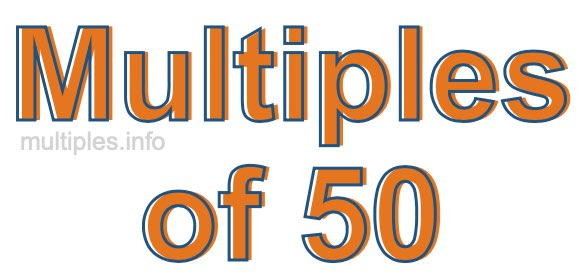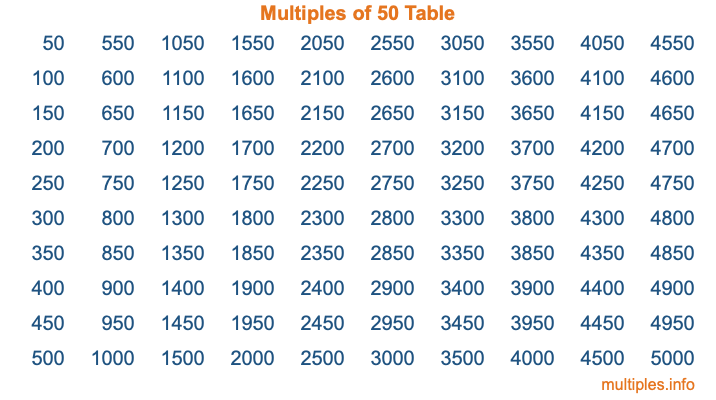Multiples of 50Welcome to the Multiples of 50 page. Here we will first teach you everything you will ever need to know about the multiples of 50, and then give you a study guide summary of everything we taught you to make sure you remember it all. Use this page to look up facts and learn information about the multiples of 50. This page will make you a multiples of fifty expert!

Definition of Multiples of 50
Multiples of 50 are all the numbers that when divided by 50 equal an integer. Each of the multiples of 50 are called a multiple. A multiple of 50 is created by multiplying 50 by an integer.

Therefore, to create a list of multiples of 50, you start with 1 multiplied by 50, then 2 multiplied by 50, then 3 multiplied by 50, and so on for as long as you want. Thus, the list of the first five multiples of 50 is 50, 100, 150, 200, and 250. To see a larger list of multiples of 50, see the printable image of Multiples of 50 further down on this page. We also have a category where you can choose any nth multiple of 50.

Multiples of 50 Checker
The Multiples of 50 Checker below checks to see if any number of your choice is a multiple of 50. In other words, it checks to see if there is any number (integer) that when multiplied by 50 will equal your number. To do that, we divide your number by 50. If the the quotient is an integer, then your number is a multiple of 50.

Is  a multiple of 50?

Least Common Multiple of 50 and ...
A Least Common Multiple (LCM) is the lowest multiple that two or more numbers have in common. This is also called the smallest common multiple or lowest common multiple and is useful to know when you are adding our subtracting fractions. Enter one or more numbers below (50 is already entered) to find the LCM.

Check out our LCM Calculator if you need more details about the Least Common Multiple or if you need the LCM for different numbers for adding and subtraction fractions.

nth Multiple of 50
As we stated above, 50 is the first multiple of 50, 100 is the second multiple of 50, 150 is the third multiple of 50, and so on. Enter a number below to find the nth multiple of 50.

th multiple of 50

Multiples of 50 vs Factors of 50
50 is a multiple of 50 and a factor of 50, but that is where the similarities end. All postive multiples of 50 are 50 or greater than 50. All positive factors of 50 are 50 or less than 50.

Below is the beginning list of multiples of 50 and the factors of 50 so you can compare:

Multiples of 50: 50, 100, 150, 200, 250, etc.

Factors of 50: 1, 2, 5, 10, 25, 50

As you can see, the multiples of 50 are all the numbers that you can divide by 50 to get a whole number. The factors of 50, on the other hand, are all the whole numbers that you can multiply by another whole number to get 50.

It's also interesting to note that if a number (x) is a factor of 50, then 50 will also be a multiple of that number (x).

Multiples of 50 vs Divisors of 50
The divisors of 50 are all the integers that 50 can be divided by evenly. Below is a list of the divisors of 50.

Divisors of 50: 1, 2, 5, 10, 25, 50

The interesting thing to note here is that if you take any multiple of 50 and divide it by a divisor of 50, you will see that the quotient is an integer.

Multiples of 50 Table
Below is an image of the first 100 multiples of 50 in a table. The table is in chronological order, column by column. The first column has the first ten multiples of 50, the second column has the next ten multiples of 50, and so on.The Multiples of 50 Table is also referred to as the 50 Times Table or Times Table of 50. You are welcome to print out our table for your studies.

Negative Multiples of 50
Although not often discussed or needed in math, it is worth mentioning that you can make a list of negative multiples of 50 by multiplying 50 by -1, then by -2, then by -3, and so on, to get the following list of negative multiples of 50:

-50, -100, -150, -200, -250, etc.

Multiples of 50 Summary
Below is a summary of important Multiples of 50 facts that we have discussed on this page. To retain the knowledge on this page, we recommend that you read through the summary and explain to yourself or a study partner why they hold true.

There are an infinite number of multiples of 50.

A multiple of 50 divided by 50 will equal a whole number.

50 divided by a factor of 50 equals a divisor of 50.

The nth multiple of 50 is n times 50.

The largest factor of 50 is equal to the first positive multiple of 50.

50 is a multiple of every factor of 50.

50 is a multiple of 50.

A multiple of 50 divided by a divisor of 50 equals an integer.

50 divided by a divisor of 50 equals a factor of 50.

Any integer times 50 will equal a multiple of 50.

Multiples of a Number
Here you can get the multiples of another number, all with the same attention to detail as we did for multiples of 50 on this page.

Multiples of
Multiples of 51
Did you find our page about multiples of fifty educational? Do you want more knowledge? Check out the multiples of the next number on our list!